# How to Calculate and Solve the Centre of Gravity of a Right Circular Cone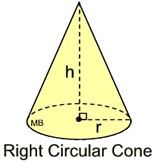This image above is a display of what a right circular cone looks like. There is only one essential parameter for calculating the centroid or centre of gravity of a right circular cone. This parameter is the height of the cone (h).

The formula for calculating the the centroid or centre of gravity of a right circular cone is:

C.G. = h / 4

As always let’s solve an example.

Find the centroid or centre of gravity of a right circular cone where the height of the cone is 12cm.
This implies that:

h = height of the cone = 12

C.G. = 12 / 4
C.G. = 3

Therefore, the centroid or centre of gravity of the right circular cone is 3.

Nickzom Calculator – The Calculator Encyclopedia is capable of calculating the centre of gravity of a right circular cone at a distance from its base measured along the vertical axis.

To get the answer and workings to center of gravity or centroid of a right circular cone using the Nickzom Calculator – The Calculator Encyclopedia. First, you need to obtain the app.

You can get this app via any of these means:

To get access to the professional version via web, you need to register and subscribe for NGN 1,500 per annum to have utter access to all functionalities.
You can also try the demo version via https://www.nickzom.org/calculator

Once, you have obtained the calculator encyclopedia app, proceed to the Calculator Map, then click on Mechanics under the Engineering section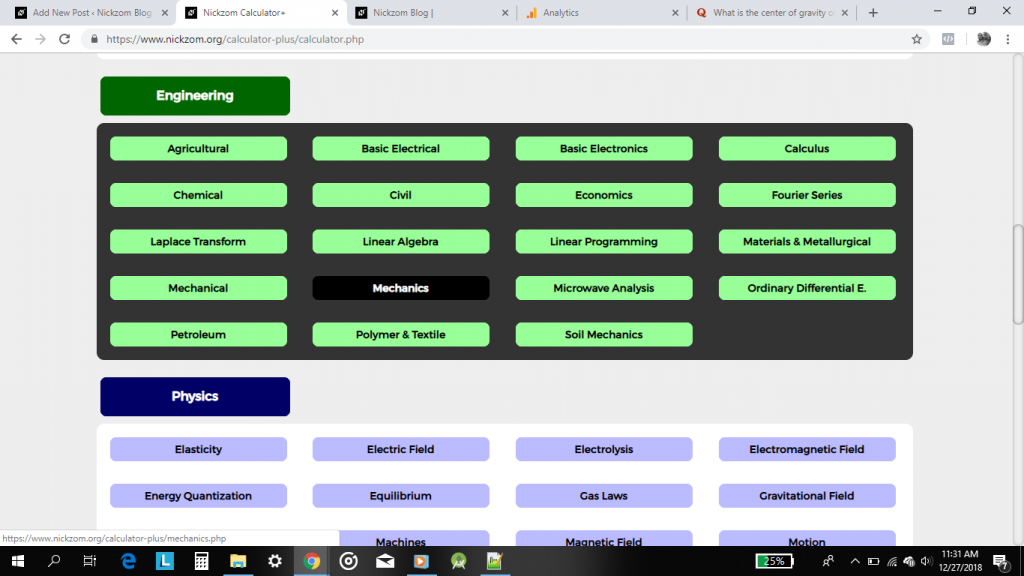Now, click on Centre of Gravity under Statics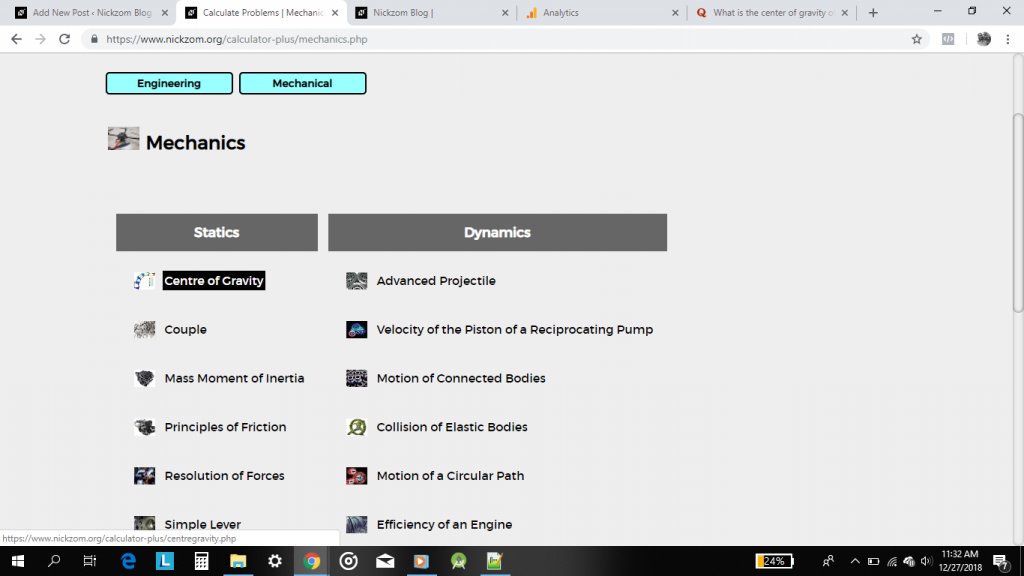Now, click on Centre of Gravity of a Right Circular Cone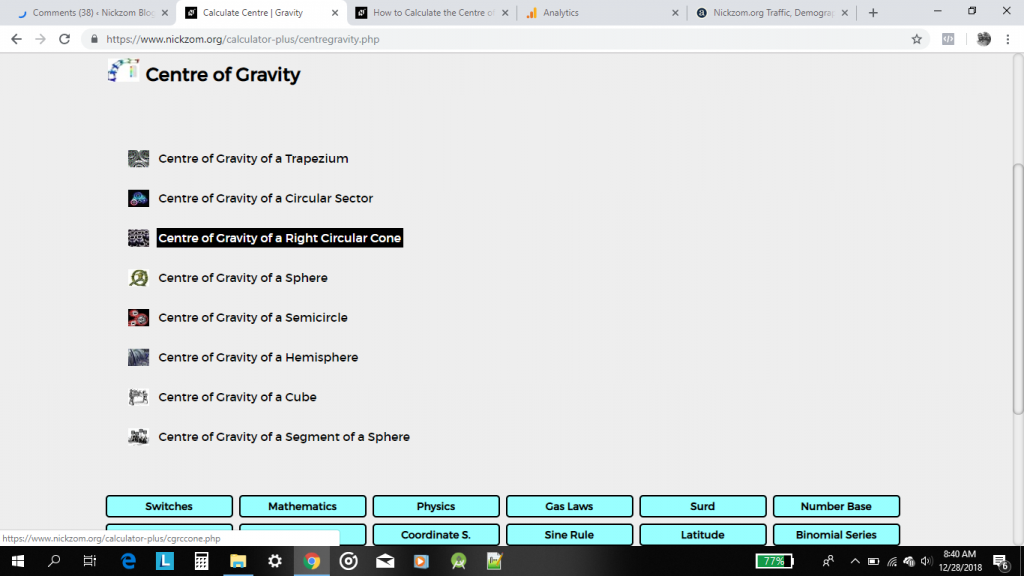The screenshot below displays the page or activity to enter your values to get answers for centre of gravity of a right circular cone according to the respective parameter which is the height of the cone (h).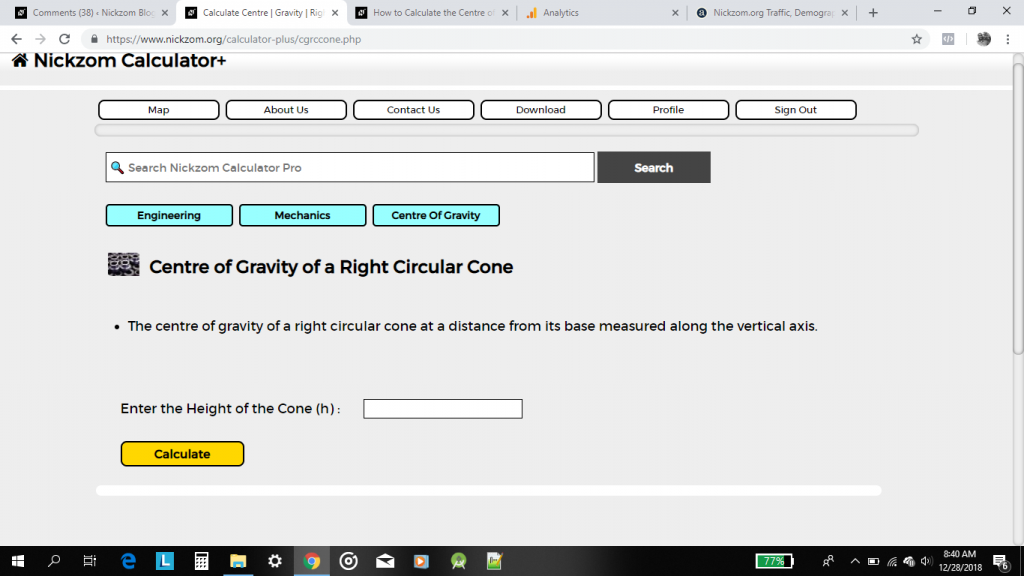Now, enter the value appropriately and accordingly for the parameter as required by the example above where the height of the cone is 12.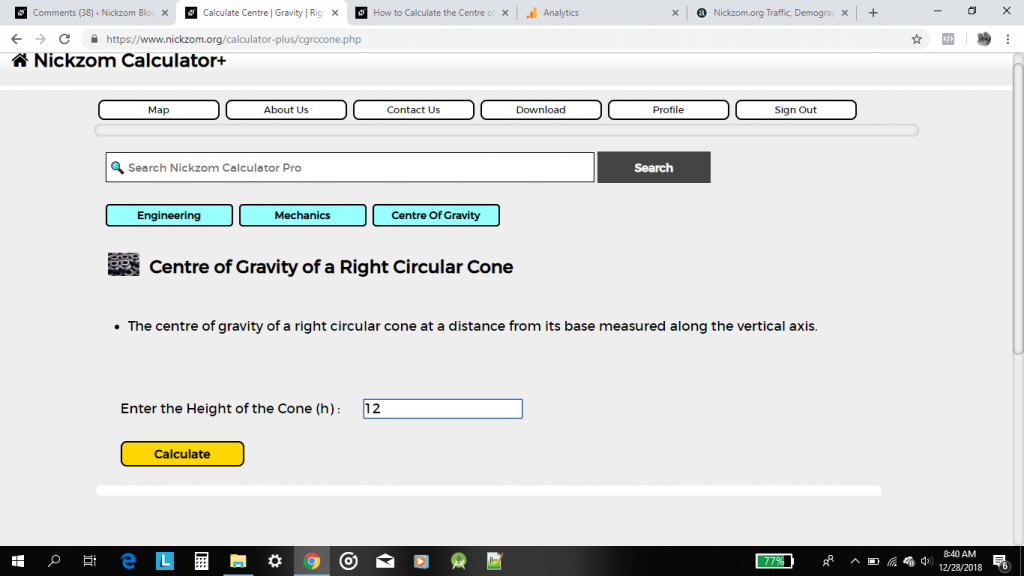Lastly, click on the Calculate button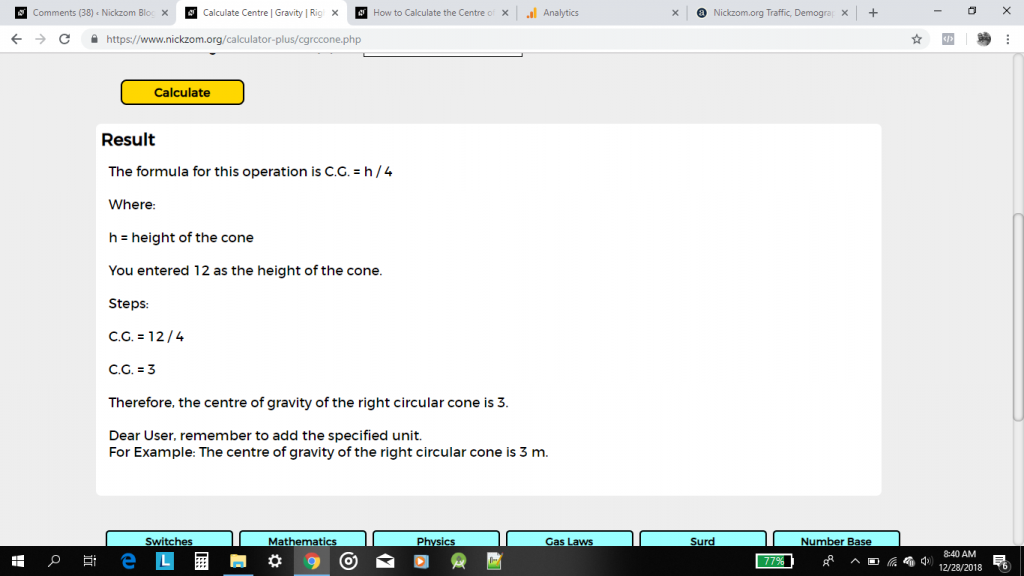As you can see from the screenshot above, Nickzom Calculator – The Calculator Encyclopedia solves for the centre of gravity or centroid of a right circular cone and presents the formula, workings and steps too.